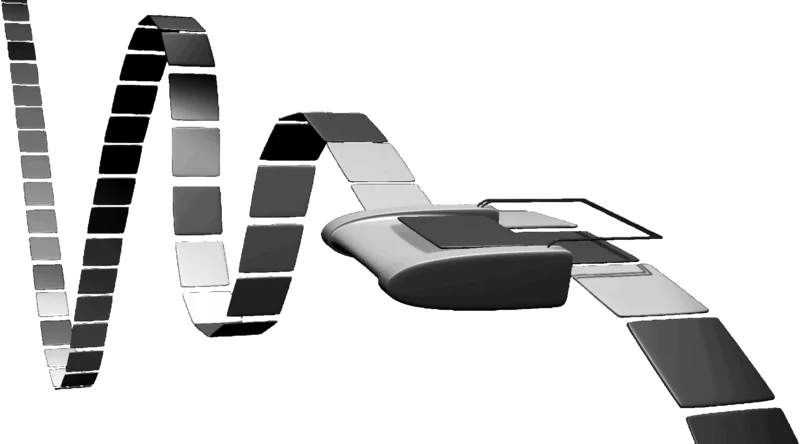## Turing MachineJust to let you know straight-away: The Turing machine is not a machine. It is a mathematical model, which was formulated by the English mathematician Alan Turing in 1936. It's a very simple model of a computer, yet it has the complete computing capability of a general purpose computer. The Turing machine (TM) serves two needs in theoretical computer science:

1. The class of languages defined by a TM, i.e. structured or recursively enumerable languages
2. The class of functions a TM is capable to compute, i.e. the partial recursive functions
A Turing machine consists only of a few components: A tape on which data can be sequentially stored. The tape consists of fields, which are sequentially arranged. Each field can contain a character of a finite alphabet. This tape has no limits, it goes on infinitely in both directions. In a real machine, the tape would have to be large enough to contain all the data for the algorithm. A TM also contains a head moving in both directions over the tape. This head can read and write one character from the field over which the head resides. The Turing machine is at every moment in a certain state, one of a finite number of states. A Turing program is a list of transitions, which determine for a given state and character ("under" the head) a new state, a character which has to be written into the field under the head and a movement direction for the head, i.e. either left, right or static (motionless).

## Formal Definition of a Turing machine

A deterministic Turing machine can be defined as a 7-tuple

M = (Q, Σ, Γ, δ, b, q0, qf)

with
• Q is a finite, non-empty set of states
• Γ is a finite, non-empty set of the tape alphabet
• Σ is the set of input symbols with Σ ⊂ Γ
• δ is a partially defined function, the transition function:
δ : (Q \ {qf}) x Γ → Q x Γ x {L,N,R}
• b ∈ &Gamma \ Σ is the blank symbol
• q0 ∈ Q is the initial state
• qf ∈ Q is the set of accepting or final states

### Example: Binary Complement function

Let's define a Turing machine, which complements a binary input on the tape, i.e. an input "1100111" e.g. will be turned into "0011000".
Σ = {0, 1}
Q = {init, final}
q0 = init
qf = final
Function Definition Description
δ(init,0) = (init, 1, R) If the machine is in state "init" and a 0 is read by the head, a 1 will be written, the state will change to "init" (so actually, it will not change) and the head will be moved one field to the right.
δ(init,1) = (init, 0, R) If the machine is in state "init" and a 1 is read by the head, a 0 will be written, the state will change to "init" (so actually, it will not change) and the head will be moved one field to the right.
δ(init,b) = (final, b, N) If a blank ("b"), defining the end of the input string, is read, the TM reaches the final state "final" and halts.

## Implementation of a Turing machine in Python

We implement a Turing Machine in Python as a class. We define another class for the read/write tape of the Turing Machine. The core of the tape inside the class Tape is a dictionary, which contains the entries of the tape. This way, we can have negative indices. A Python list is not a convenient data structure, because Python lists are bounded on one side, i.e. bounded by 0.

We define the method str(self) for the class Tape. str(self) is called by the built-in str() function. The print function uses also the str function to calculate the "informal" string representation of an object, in our case the tape of the TM. The method get_tape() of our class TuringMachine makes use of the str representation returned by str.

With the aid of the method getitem(), we provide a reading access to the tape via indices. The definition of the method setitem() allows a writing access as well, as we can see e.g. in the statement
self.__tape[self.__head_position] = y
of our class TuringMachine implementation.

class Tape(object):

blank_symbol = " "

def __init__(self,
tape_string = ""):
self.__tape = dict((enumerate(tape_string)))
# last line is equivalent to the following three lines:
#self.__tape = {}
#for i in range(len(tape_string)):
#    self.__tape[i] = input[i]

def __str__(self):
s = ""
min_used_index = min(self.__tape.keys())
max_used_index = max(self.__tape.keys())
for i in range(min_used_index, max_used_index):
s += self.__tape[i]
return s

def __getitem__(self,index):
if index in self.__tape:
return self.__tape[index]
else:
return Tape.blank_symbol

def __setitem__(self, pos, char):
self.__tape[pos] = char

class TuringMachine(object):

def __init__(self,
tape = "",
blank_symbol = " ",
initial_state = "",
final_states = None,
transition_function = None):
self.__tape = Tape(tape)
self.__blank_symbol = blank_symbol
self.__current_state = initial_state
if transition_function == None:
self.__transition_function = {}
else:
self.__transition_function = transition_function
if final_states == None:
self.__final_states = set()
else:
self.__final_states = set(final_states)

def get_tape(self):
return str(self.__tape)

def step(self):
if x in self.__transition_function:
y = self.__transition_function[x]
if y == "R":
elif y == "L":
self.__current_state = y

def final(self):
if self.__current_state in self.__final_states:
return True
else:
return False


Using the TuringMachine class:

initial_state = "init",
accepting_states = ["final"],
transition_function = {("init","0"):("init", "1", "R"),
("init","1"):("init", "0", "R"),
("init"," "):("final"," ", "N"),
}
final_states = {"final"}

t = TuringMachine("010011001 ",
initial_state = "init",
final_states = final_states,
transition_function=transition_function)

print("Input on Tape:\n" + t.get_tape())

while not t.final():
t.step()

print("Result of the Turing machine calculation:")
print(t.get_tape())

Input on Tape:
010011001
Result of the Turing machine calculation:
101100110